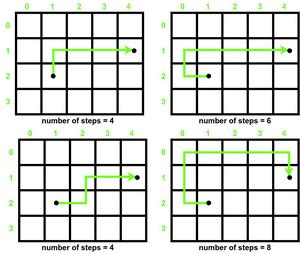# Check if it is possible to reach destination in even number of steps in an Infinite Matrix

• Difficulty Level : Medium
• Last Updated : 01 Sep, 2021

Given a source and destination in a matrix[][] of infinite rows and columns, the task is to find whether it is possible to reach the destination from the source in an even number of steps. Also, you can only move up, down, left, and right.

Examples:

Attention reader! Don’t stop learning now. Get hold of all the important DSA concepts with the DSA Self Paced Course at a student-friendly price and become industry ready.  To complete your preparation from learning a language to DS Algo and many more,  please refer Complete Interview Preparation Course.

In case you wish to attend live classes with experts, please refer DSA Live Classes for Working Professionals and Competitive Programming Live for Students.

Input: Source = {2, 1}, Destination = {1, 4}
Output: Yes

Input: Source = {2, 2}, Destination = {1, 4}
Output: No

Observation:

The observation is that if the steps required to reach the destination in the shortest path is even, then steps required in every other route to reach it will always be even. Also, there can be an infinite number of ways to reach the target point. Some paths to reach (4, 1) from (1, 2) in a 4 x 5 matrix are given below :Minimum number of steps required =4

So our problem is reduced in finding the minimum number of steps required to reach the destination from source in a matrix, which can be calculated easily by simply taking the sum of the absolute values of the difference between the X coordinates and Y coordinates.Below is the implementation of the above approach:

## C++

 `// C++ Program for the above approach` `#include ` `using` `namespace` `std;`   `// Function to check destination can be` `// reached from source in even number of` `// steps` `void` `IsEvenPath(``int` `Source[], ``int` `Destination[])` `{` `    ``// Coordinates differences` `    ``int` `x_dif = ``abs``(Source - Destination);` `    ``int` `y_dif = ``abs``(Source - Destination);`   `    ``// minimum number of steps required` `    ``int` `minsteps = x_dif + y_dif;`   `    ``// Minsteps is even` `    ``if` `(minsteps % 2 == 0)` `        ``cout << ``"Yes"``;`   `    ``// Minsteps is odd` `    ``else` `        ``cout << ``"No"``;` `}`   `// Driver Code` `int` `main()` `{` `    ``// Given Input` `    ``int` `Source[] = { 2, 1 };` `    ``int` `Destination[] = { 1, 4 };`   `    ``// Function Call` `    ``IsEvenPath(Source, Destination);`   `    ``return` `0;` `}`

## Java

 `// Java program for the above approach` `import` `java.lang.*;` `import` `java.util.*;`   `class` `GFG{`   `// Function to check destination can be` `// reached from source in even number of` `// steps` `static` `void` `IsEvenPath(``int` `Source[], ``int` `Destination[])` `{` `    `  `    ``// Coordinates differences` `    ``int` `x_dif = Math.abs(Source[``0``] - Destination[``0``]);` `    ``int` `y_dif = Math.abs(Source[``1``] - Destination[``1``]);`   `    ``// Minimum number of steps required` `    ``int` `minsteps = x_dif + y_dif;`   `    ``// Minsteps is even` `    ``if` `(minsteps % ``2` `== ``0``)` `        ``System.out.println(``"Yes"``);`   `    ``// Minsteps is odd` `    ``else` `        ``System.out.println(``"No"``);` `}`   `// Driver code` `public` `static` `void` `main(String[] args)` `{` `    `  `    ``// Given Input` `    ``int` `Source[] = { ``2``, ``1` `};` `    ``int` `Destination[] = { ``1``, ``4` `};`   `    ``// Function Call` `    ``IsEvenPath(Source, Destination);` `}` `}`   `// This code is contributed by sanjoy_62`

## Python3

 `# Python3 program for the above approach`   `# Function to check destination can be` `# reached from source in even number of` `# steps` `def` `IsEvenPath(Source, Destination):`   `    ``# Coordinates differences` `    ``x_dif ``=` `abs``(Source[``0``] ``-` `Destination[``0``])` `    ``y_dif ``=` `abs``(Source[``1``] ``-` `Destination[``1``])`   `    ``# Minimum number of steps required` `    ``minsteps ``=` `x_dif ``+` `y_dif`   `    ``# Minsteps is even` `    ``if` `(minsteps ``%` `2` `=``=` `0``):` `        ``print``(``"Yes"``)`   `    ``# Minsteps is odd` `    ``else``:` `        ``print``(``"No"``)`   `# Driver Code` `if` `__name__ ``=``=` `'__main__'``:` `    `  `    ``# Given Input` `    ``Source ``=` `[ ``2``, ``1` `]` `    ``Destination ``=` `[ ``1``, ``4` `]`   `    ``# Function Call` `    ``IsEvenPath(Source, Destination)`   `# This code is contributed by mohit kumar 29`

## C#

 `// C# program for the above approach` `using` `System;`   `class` `GFG{`   `// Function to check destination can be` `// reached from source in even number of` `// steps` `static` `void` `IsEvenPath(``int``[] Source, ``int``[] Destination)` `{` `    `  `    ``// Coordinates differences` `    ``int` `x_dif = Math.Abs(Source - Destination);` `    ``int` `y_dif = Math.Abs(Source - Destination);`   `    ``// Minimum number of steps required` `    ``int` `minsteps = x_dif + y_dif;`   `    ``// Minsteps is even` `    ``if` `(minsteps % 2 == 0)` `        ``Console.WriteLine(``"Yes"``);`   `    ``// Minsteps is odd` `    ``else` `        ``Console.WriteLine(``"No"``);` `}`   `// Driver code` `public` `static` `void` `Main(``string``[] args)` `{` `    ``// Given Input` `    ``int``[] Source = { 2, 1 };` `    ``int``[] Destination = { 1, 4 };`   `    ``// Function Call` `    ``IsEvenPath(Source, Destination);` `}` `}`   `// This code is contributed by code_hunt.`

## Javascript

 ``

Output

`Yes`

Time Complexity: O(1)
Auxiliary Space: O(1)

My Personal Notes arrow_drop_up
Recommended Articles
Page :